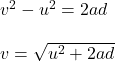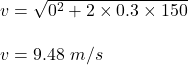## A truck starts from rest with an acceleration of 0.3 m/ S^2 find its speed in km/h when it has moves through 150 m​

Question

A truck starts from rest with an acceleration of 0.3 m/ S^2 find its speed in km/h when it has moves through 150 m​

in progress 0
2 months 2021-07-28T22:15:21+00:00 1 Answers 3 views 0

v = 34.128 km/hr

Explanation:

Given that,

The initial speed of a truck, u = 0

Acceleration of the truck, a = 0.3 m/s²

Distance moved, d = 150 m

Let the final speed of the truck is v. Using third equation of motion i.e.Put all the values,or

v = 34.128 km/h

So, the final speed of the truck is equal to 34.128 km/h.